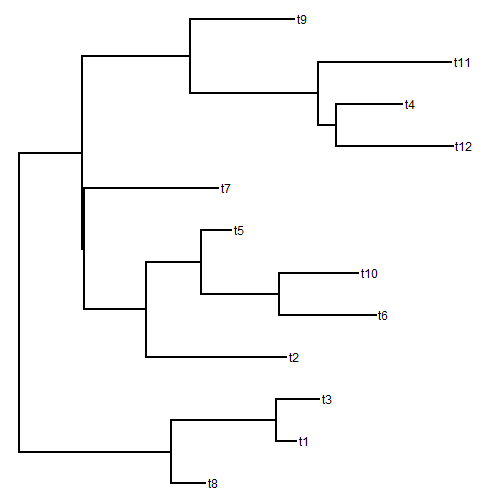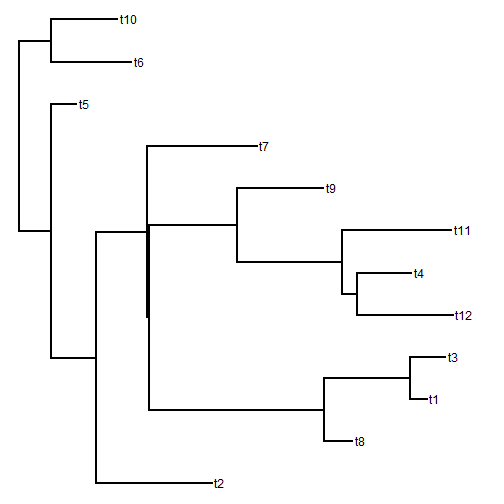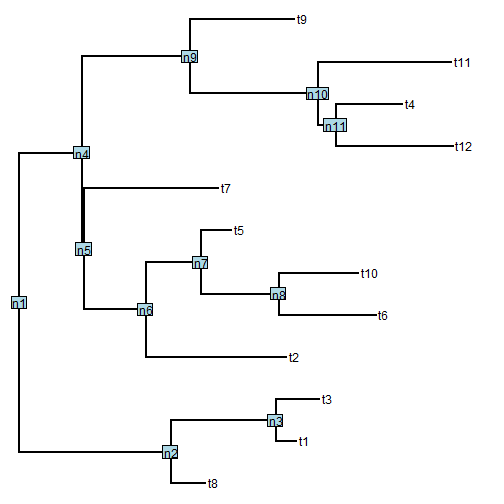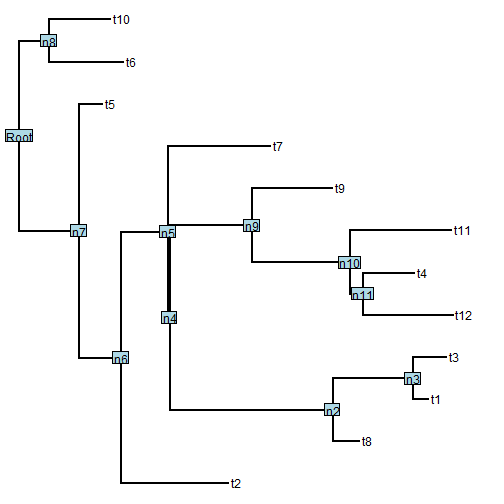## Tuesday, February 10, 2015

### Bug fix for reroot for trees with node labels

A phytools user last night very helpfully reported that the phytools function `reroot`, which reroots the tree along an edge, throws an error if the input tree has node labels. Indeed, this seems to be the case. Here is the code supplied by the user (slightly modified) demonstrating the bug:

``````library(phytools)
set.seed(1) ## just for reproducibility
##  no node labels
tr<-rtree(12)
plotTree(tr)
````````````nod<-fastMRCA(tr,"t6","t10") ## arbitrarily
rtr<-reroot(tr,node.number=nod,position=0.5*tr\$edge.length[which(tr\$edge[,2]==nod)])
plotTree(rtr) ## works fine
````````````## now try the same with node labels
tr\$node.label<-paste("n",1:tr\$Nnode,sep="")
plotTree(tr)
nodelabels(tr\$node.label)
````````````rtr<-reroot(tr, node.number=nod, position=0.5*tr\$edge.length[which(tr\$edge[,2]==nod)])
``````
``````## Error in if (newroot == ROOT) {: argument is of length zero
``````

This morning I was able to get to the bottom of this bug. Basically, the function `reroot` uses the phytools (mostly internal, but in the namespace) function `splitTree` to first split the tree at the desired point for re-rooting, then reroots the tree using the split point in the “parent” subtree as the new root. It identifies this split point in the parent tree because it has the tip label of `"NA"`. Unfortunately, if the input tree has node labels, then the new node will be labeled not with `"NA"`, but with the node label of the input tree.

I have now posted a new version of this function with this bug fixed. I accomplished this by checking for tips labeled first with `"NA"` and then, if none are found, with any of the node labels of the input tree. This will work fine unless any of the node labels are the same as tip labels (or `"NA"`). This probably should be avoided anyway.

Here is how it works:

``````detach("package:phytools",unload=TRUE)
## install new phytools with bug fix
install.packages("phytools_0.4-47.tar.gz",type="source",repos=NULL)
``````
``````## Installing package into 'C:/Users/Liam/Documents/R/win-library/3.1'
## (as 'lib' is unspecified)
``````
``````library(phytools)
packageVersion("phytools")
``````
``````##  '0.4.47'
``````
``````rtr<-reroot(tr, node.number=nod, position=0.5*tr\$edge.length[which(tr\$edge[,2]==nod)])
plotTree(rtr)
nodelabels(rtr\$node.label)
``````Great!